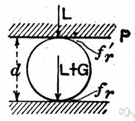# coefficient

(redirected from coefficient of nondetermination)
Also found in: Thesaurus, Medical, Encyclopedia.

## co·ef·fi·cient

(kō′ə-fĭsh′ənt)
n.
1. A number or symbol multiplied with a variable or an unknown quantity in an algebraic term, as 4 in the term 4x, or x in the term x(a + b).
2. A numerical measure of a physical or chemical property that is constant for a system under specified conditions, such as the coefficient of friction.

## coefficient

(ˌkəʊɪˈfɪʃənt)
n
1. (Mathematics) maths
a. a numerical or constant factor in an algebraic term: the coefficient of the term 3xyz is 3.
b. the product of all the factors of a term excluding one or more specified variables: the coefficient of x in 3axyz is 3ayz.
2. (General Physics) physics a value that relates one physical quantity to another
[C17: from New Latin coefficiēns, from Latin co- together + efficere to effect]
Collins English Dictionary – Complete and Unabridged, 12th Edition 2014 © HarperCollins Publishers 1991, 1994, 1998, 2000, 2003, 2006, 2007, 2009, 2011, 2014

## co•ef•fi•cient

(ˌkoʊ əˈfɪʃ ənt)

n.
1. a number or quantity placed generally before and multiplying another quantity, as 3 in the expression 3x.
2. Physics. a constant that is a measure of a property of a substance, body, or process: coefficient of friction.
3. acting in consort; cooperating.
[1655–65; < New Latin coefficient-, s. of coefficiēns. See co-, efficient]

## co·ef·fi·cient

(kō′ə-fĭsh′ənt)
A number or symbol multiplied with a variable or an unknown quantity in an algebraic term. For example, 4 is the coefficient in the term 4x, and x is the coefficient in x(a + b).
ThesaurusAntonymsRelated WordsSynonymsLegend:
 Noun 1coefficient - a constant number that serves as a measure of some property or characteristicconstant - a number representing a quantity assumed to have a fixed value in a specified mathematical context; "the velocity of light is a constant"absorptance, absorption coefficient, coefficient of absorption - a measure of the rate of decrease in the intensity of electromagnetic radiation (as light) as it passes through a given substance; the fraction of incident radiant energy absorbed per unit mass or thickness of an absorber; "absorptance equals 1 minus transmittance"coefficient of drag, drag coefficient - the ratio of the drag on a body moving through air to the product of the velocity and the surface area of the bodycoefficient of friction - the ratio of the weight of an object being moved along a surface and the force that maintains contact between the object and the surfacecoefficient of mutual induction, mutual inductance - a measure of the induction between two circuits; the ratio of the electromotive force in a circuit to the corresponding change of current in a neighboring circuit; usually measured in henriescoefficient of self induction, self-inductance - the ratio of the electromotive force produced in a circuit by self-induction to the rate of change of current producing it, expressed in henriesmodulus - (physics) a coefficient that expresses how much of a specified property is possessed by a specified substancecoefficient of expansion, expansivity - the fractional change in length or area or volume per unit change in temperature at a given constant pressurecoefficient of reflection, reflectance, reflection factor, reflectivity - the fraction of radiant energy that is reflected from a surfacetransmittance, transmission - the fraction of radiant energy that passes through a substanceabsolute viscosity, coefficient of viscosity, dynamic viscosity - a measure of the resistance to flow of a fluid under an applied forceweighting, weight - (statistics) a coefficient assigned to elements of a frequency distribution in order to represent their relative importance
Based on WordNet 3.0, Farlex clipart collection. © 2003-2012 Princeton University, Farlex Inc.
Translations
koeficientsoučinitel
koefficient
kerroinmyötävaikuttava
együttható

## coefficient

[ˌkəʊɪˈfɪʃənt] N
Collins Spanish Dictionary - Complete and Unabridged 8th Edition 2005 © William Collins Sons & Co. Ltd. 1971, 1988 © HarperCollins Publishers 1992, 1993, 1996, 1997, 2000, 2003, 2005

## coefficient

[ˌkəʊɪˈfɪʃənt] n
Collins English/French Electronic Resource. © HarperCollins Publishers 2005

## coefficient

n (Math, Phys) → Koeffizient m
Collins German Dictionary – Complete and Unabridged 7th Edition 2005. © William Collins Sons & Co. Ltd. 1980 © HarperCollins Publishers 1991, 1997, 1999, 2004, 2005, 2007

## coefficient

[ˌkəʊɪˈfɪʃnt] n
Collins Italian Dictionary 1st Edition © HarperCollins Publishers 1995

## co·ef·fi·cient

n. coeficiente, indicación de cambios físicos o químicos producidos por variantes de ciertos factores.
English-Spanish Medical Dictionary © Farlex 2012
Site: Follow: Share:
Open / Close